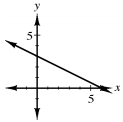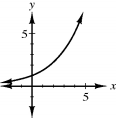### Home > ACC7 > Chapter 9 Unit 10 > Lesson CC3: 9.1.4 > Problem9-46

9-46.

Sketch an example of each type of graph described below.

1. linear and decreasing

Graph a line with the formula $y = mx + b$, where m is negative.

Sketch a line on a graph that goes down as it goes right.

An example of a decreasing linear graph is shown with the equation $y = −2x + 6$1. non-linear and increasing

Graph a relationship where either $x$ or $y$ has an exponent greater than $1$.

An equation in which $x$ or $y$ has an exponent greater than one will create a curve, rather than a line.

An example of an increasing, non-linear graph is shown with the curve with the equation $y=3^x$.